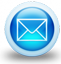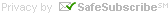Types of Construction Estimates

Services » Types of Construction Estimates

Numerous estimating techniques prevail in the market and these can be categorized in the following ways :-

• Conceptual Estimates
• Detailed estimates

Conceptual Estimates

Basic parametric sizes are used for the type of building to reach an order of magnitude estimate. Quantitites do not play a large role. Historical Ratios are used to establish a cost per unit. Basic scopes for trades are established.

Detailed Estimate

A detailed estimate is the most accurate form of estimate. It is made when the scope of work is clearly described and the detailed design is underway in order that the crucial features of the facility are identifiable. This type of estimate is set up to find out the quantities and costs of everything for a project. These types of estimates help contractor to complete a construction successfully. A detailed estimate is arranged in the following two ways :-

• Unit quantity method
• Total quantity method

Unit Quantity Method

In this method, the work is segregated into as many operations or items as per requirements. A unit of measurement is settled on. The total quantity of work under each item is extracted in the suitable unit of measurement. Every item is evaluated and exercised with the total cost per unit quantity. After that the cost per unit quantity is multiplied by the number of units to determine the total cost for the item. As for instance, at the time of preparing the cost of a building work, the quantity of brickwork in the building is gauged by cubic meters. The total cost along with cost of materials. labor, plant, overheads and profit per cubic meter of brickwork is generated and after that the predictable cost of brickwork is calculated by multiplying this unit cost by the number of cubic meters of brickwork in the building.

The benefit of this method is that the unit costs on different jobs are instantly comparable and that the total estimate can be rectified effortlessly for variations in quantities.

Total Quantity Method

An item of work is separated with the following five subdivisions In the total quantity method:

• Materials
• Labor
• Plant
• Profit

The total quantities of each kind or class of material or labor are established and multiplied by their individual unit cost. The cost of plant, overhead expenses and profit are decided In the same way. The costs of all the five sub-heads are added to provide the estimated cost of the item of work.Estimating Sheets